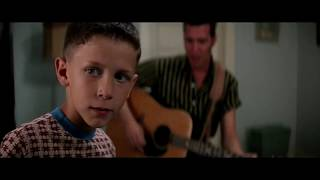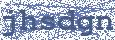Wilkinson - Run (feat. Jem Cooke)

## WILKINSON

Run Lyrics (feat. Jem Cooke)

I wander slowly past your door
Haven't been back here for a while now
We kissed a thousand times before
Do you remember?

You turn my hand and let me off
As if we only had forever
And we shone brighter than the sun
Do you remember?

How we would run
Like the tears I cried today, we would run
Like the rain falls down my face, we would run
Like the blood inside my veins, we would run
We would run, we would run

We would run, run, run
We would run, run, run
We would run, run, run
We would run, run, run
We would run, run, run
We would run, run, run
We would run, run, run
We would run, run, run

But now I check this path alone
I wish I had a way to take it back
Back to the place I call my home
I still remember

You turn my hand and let me off
As if we only had forever
And we shone brighter than the sun
Do you remember?

How we would run
Like the tears I cried today, we would run
Like the rain falls down my face, we would run
Like the blood inside my veins, we would run
We would run, we would run

We would run, run, run
We would run, run, run
We would run, run, run
We would run, run, run
We would run, run, run
We would run, run, run
We would run, run, run
We would run, run, run

We would run
We would run
We would run
We would runHottest Lyrics with Videos
98466838bb155405d110dabaa5821a15